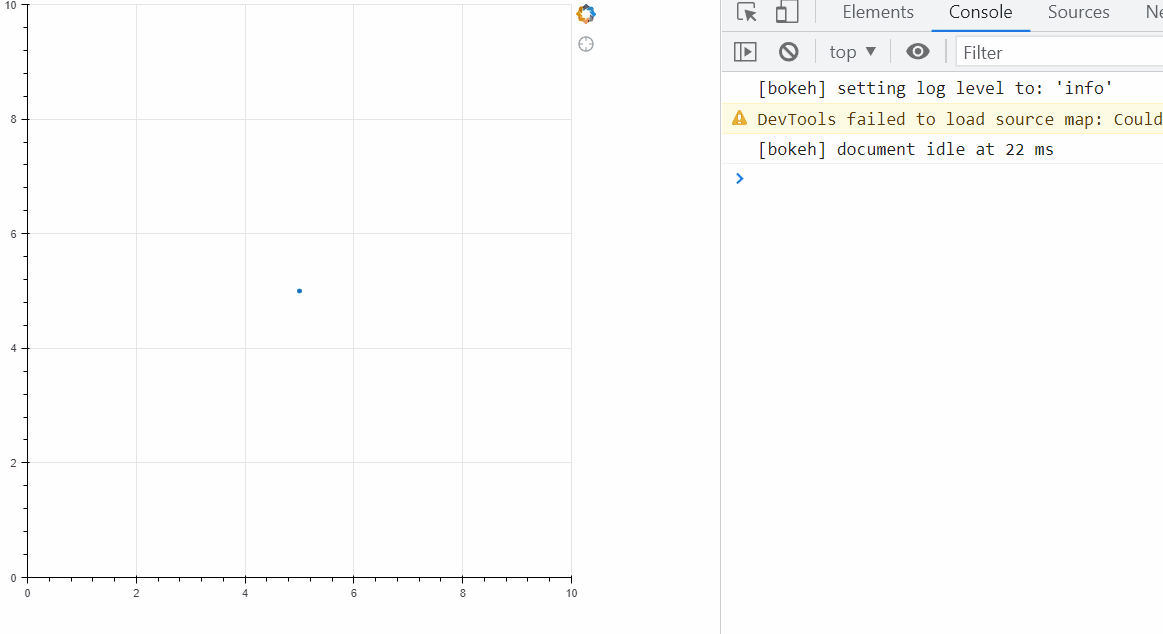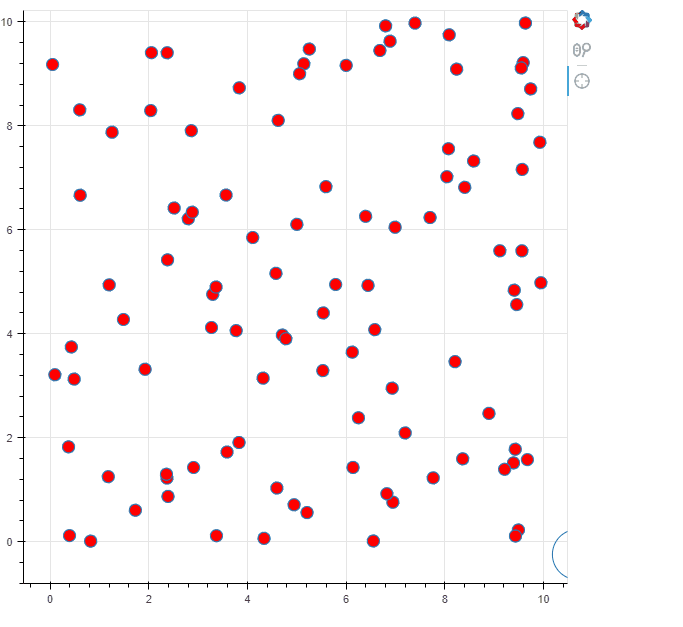# Custom Tool listening for multiple events

### What are you trying to do?

Dip my toes into developing a custom tool (tentatively calling it CircleTool). I’m very closely following this → A new custom tool — Bokeh 2.4.3 Documentation . CircleTool takes a CDS containing x,y and r fields.

What I want to have happen when “CircleTool” is active:

→ When user does a mouse wheel/scroll: adjust the ‘r’ field in its CDS. I have this working great.
→ When user moves over the canvas: update the x and y fields in its CDS. I can’t get this to work!

### What have you tried that did NOT work as expected?

The issue is I can’t seem to get the MoveEvent to trigger. I’ve followed (what I think) is logical (I even looked at the crosshair tool.ts code here to try and model my attempt here off it: bokeh/crosshair_tool.ts at branch-3.0 · bokeh/bokeh · GitHub but it just simply isn’t happening.

Code:

``````# -*- coding: utf-8 -*-

from bokeh.core.properties import Instance
from bokeh.io import output_file, show
from bokeh.models import ColumnDataSource, Tool, CustomJS
from bokeh.plotting import figure
from bokeh.util.compiler import TypeScript

TS_CODE = """
import {GestureTool, GestureToolView} from "models/tools/gestures/gesture_tool"
import * as p from "core/properties"
import {ScrollEvent, MoveEvent} from "core/ui_events"
import {ColumnDataSource} from "models/sources/column_data_source"

export class CircleToolView extends GestureToolView {
model: CircleTool

//this is executed on scroll
_scroll(ev: ScrollEvent): void {
if (ev.delta<0){
this.model.source.data.r = this.model.source.data.r - (this.model.source.data.r*.05)}
else {this.model.source.data.r = this.model.source.data.r*1.05}
console.log(this.model.source.data.r)
}

//why does this not execute on mousemove?
_move(ev: MoveEvent): void {
console.log('Do Something!')
console.log(ev)
}
}

export namespace CircleTool {
export type Attrs = p.AttrsOf<Props>

export type Props = GestureTool.Props & {
source: p.Property<ColumnDataSource>
}
}

export interface CircleTool extends CircleTool.Attrs {}

export class CircleTool extends GestureTool {
properties: CircleTool.Props
__view_type__: CircleToolView

constructor(attrs?: Partial<CircleTool.Attrs>) {
super(attrs)
}

tool_name = "Circle Tool"
icon = "bk-tool-icon-crosshair"
event_type = "scroll" as "scroll"
default_order = 12

static {
this.prototype.default_view = CircleToolView

this.define<CircleTool.Props>(({Ref}) => ({
source: [ Ref(ColumnDataSource) ],
}))
}
}
"""

class CircleTool(Tool):
__implementation__ = TypeScript(TS_CODE)
source = Instance(ColumnDataSource)

source = ColumnDataSource(data=dict(x=, y=, r=))

t = CircleTool(source=source)

plot = figure(x_range=(0, 10), y_range=(0, 10), tools=[t])

plot.scatter('x', 'y', source=source)
# plot.js_on_event('tap',CustomJS(args=dict(t=t),code='''console.log(t)''')) #looking at stuff
show(plot)
``````

gif showing the ScrollEvent working like a dream but the MoveEvent not:What am I missing here?!

The tool only registers for the scroll event:

``````event_type = "scroll" as "scroll"
``````

Presumably you want:

``````event_type = ["scroll" as "scroll", "move" as "move"]
``````
1 Like

Thank you! I played with that part too, could not figure out how to structure it.

Took me a while to figure out how to translate sx and sy into data units, but here it isOverall idea was to define a tool that when enabled would move around a circle, and the radius of that circle can be adjusted via scroll. Then with some basic circle math in CustomJS you can select items that live in that circle.

Working code:

``````from bokeh.core.properties import Instance
from bokeh.io import output_file, show
from bokeh.models import ColumnDataSource, Tool, CustomJS
from bokeh.plotting import figure, save
from bokeh.util.compiler import TypeScript
import numpy as np

TS_CODE = """
import {GestureTool, GestureToolView} from "models/tools/gestures/gesture_tool"
import * as p from "core/properties"
import {ScrollEvent, MoveEvent} from "core/ui_events"
import {ColumnDataSource} from "models/sources/column_data_source"

export class CircleToolView extends GestureToolView {
model: CircleTool

//this is executed on scroll
_scroll(ev: ScrollEvent): void {
if (ev.delta<0){
var new_r = this.model.source.data.r - (this.model.source.data.r*.05)}
else {var new_r = this.model.source.data.r*1.05}

const newdata = {'x':[this.model.source.data.x],'y':[this.model.source.data.y],'r':[new_r]}
this.model.source.stream(newdata,1)
this.model.source.change.emit()
}

//this executes on mousemove
_move(ev: MoveEvent): void {
const sbox = this.plot_view.frame.bbox
//get data x
const xr = this.plot_view.axis_views.computed_bounds
const mx = (xr-xr)/(sbox.x1-sbox.x0)
const bx = xr-(mx*sbox.x0)
const x = ev.sx*mx+bx

//get data y
//sy is inverted
const yr = this.plot_view.axis_views.computed_bounds
const my = (yr-yr)/(sbox.y0-sbox.y1)
const by = yr-(my*sbox.y1)

const y = ev.sy*my+by

const newdata = {'x':[x],'y':[y],'r':[this.model.source.data.r]}
this.model.source.stream(newdata,1)

}
}

export namespace CircleTool {
export type Attrs = p.AttrsOf<Props>

export type Props = GestureTool.Props & {
source: p.Property<ColumnDataSource>
}
}

export interface CircleTool extends CircleTool.Attrs {}

export class CircleTool extends GestureTool {
properties: CircleTool.Props
__view_type__: CircleToolView

constructor(attrs?: Partial<CircleTool.Attrs>) {
super(attrs)
}

tool_name = "Circle Tool"
icon = "bk-tool-icon-crosshair"
event_type = ["scroll" as "scroll", "move" as "move"]
default_order = 12

static {
this.prototype.default_view = CircleToolView

this.define<CircleTool.Props>(({Ref}) => ({
source: [ Ref(ColumnDataSource) ],
}))
}
}
"""

class CircleTool(Tool):
__implementation__ = TypeScript(TS_CODE)
source = Instance(ColumnDataSource)

source = ColumnDataSource(data=dict(x=, y=, r=[0.5]))
t = CircleTool(source=source)

dsrc = ColumnDataSource(data={'x':np.random.random(100)*10,'y':np.random.random(100)*10})

plot = figure(x_range=(0, 10), y_range=(0, 10), tools=[t,'wheel_zoom'])

r = plot.circle(x='x', y='y', radius='r', source=source,fill_alpha=0)
dr = plot.scatter(x='x',y='y',source=dsrc,fill_color='red',size=12)

cb = CustomJS(args=dict(source=source,dsrc=dsrc)
,code='''
const x = source.data.x
const y = source.data.y
const r = source.data.r
var sel_inds = []
for (var i=0;i<dsrc.data.x.length;i++){
var xp = dsrc.data.x[i]
var yp = dsrc.data.y[i]
if ( ((xp-x)**2+(yp-y)**2)**0.5 < r ){
sel_inds.push(i)}
}
dsrc.selected.indices = sel_inds
dsrc.change.emit()
''')
source.js_on_change('streaming',cb)
save(plot,'test.html')
``````The baller move here would be to abstract this further to do this kind of action on arbitrary glyphs (so SquareTool, RectTool, EllipseTool (even with a rotation… /lol shudder) and to do the selection within the TS code, and have it act only specific renderers as supplied upon instantiation (like HoverTools do). But yeah, I’m not there yet (like not even close), but this is a solid first step.

Man, going through this exercise just brings on a whole new level of respect for what you’ve put together with this library. Thanks.

1 Like

To be fair there’s no docs about this anywhere, I had to go look at at source for the the multi-gesture edit tools to see what they do.

Man, going through this exercise just brings on a whole new level of respect for what you’ve put together with this library.

Thanks! This is a really cool example you’ve made. We are still not where I’d like to be for BokehJS / extension stability, but I hope after 3.0 comes out we are a bit closer.

This topic was automatically closed 90 days after the last reply. New replies are no longer allowed.